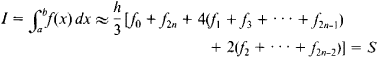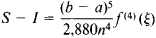# Simpson's Rule

(redirected from Composite simpson's rule)

## Simpson's rule

[′sim·sənz ‚rül]
(mathematics)
Also known as parabolic rule.
A basic approximation formula for definite integrals which states that the integral of a real-valued function ƒ on an interval [a,b ] is approximated by h [ƒ(a) + 4ƒ(g + h) + ƒ(b)]/3, where h = (b-a)/2; this is the area under a parabola which coincides with the graph of ƒ at the abscissas a, a + h, and b.
A method of approximating a definite integral over an interval which is equivalent to dividing the interval into equal subintervals and applying the formula in the first definition to each subinterval.
(petroleum engineering)
A mathematical relationship for calculating the oil- or gas-bearing net-pay volume of a reservoir; uses the contour lines from a subsurface geological map of the reservoir, including gas-oil and gas-water contacts.

## Simpson’s Rule

a formula for approximating definite integrals. In has the formwhere h = (b - a)/2n and fi = f(a + ih), i = 0, 1, 2,…, 2n.

The derivation of Simpson’s rule is based on the replacement of the integrand f(x) on each of the closed intervals [a + 2hk, a + 2h(k + 1)], k = 0, 1,…, n - 1, by a corresponding interpolation polynomial of the second degree (seeINTERPOLATION FORMULAS). In geometric terms, the curve described by the equation y = f(x) is replaced by an approximating curve consisting of segments of parabolas. The error resulting from the use of Simpson’s rule iswhere aξb. If f(x) is a polynomial of degree m ≤ 3, then Simpson’s rule is not approximate but exact, since in this case f(4)(x) = 0.

Simpson’s rule is named after T. Simpson, who devised it in 1743. The rule was, however, known earlier. For example, it was given by J. Gregory in 1668.

Other formulas for the approximate calculation of definite integrals are discussed in the article APPROXIMATE INTEGRATION.

Mentioned in ?
References in periodicals archive ?
(ii) For K(x, s) [not equal to] 1, composite Simpson's rule is employed for solving the integral part:
This sort of approach is termed the composite Simpson's rule and is denoted by the following (Jeffrey & Dai, 1995, p.364; Wikipedia, 2012; Chapra, 2005, p.475):
In this paper, we combined PSO and composite Simpson's rule for the optimization of a complex inventory model.
Clearly, the best fitted line equation (3.22) shows that the error in the composite Simpson's rule is of order M = 4 and the error constant is
Four examples were selected and presented to illustrate the approach including Taylor polynomial approximation, the 5-point forward numerical differentiation formula, the composite Simpson's rule and the 4-stage explicit Runge-Kutta method.

Site: Follow: Share:
Open / Close